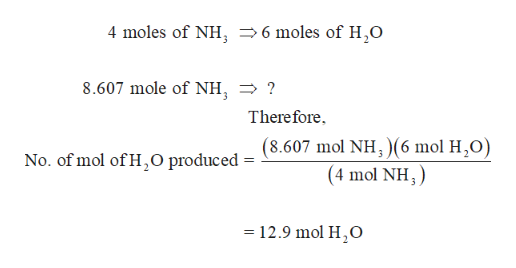# Determine the number of moles of H2O produced when o8.607 mole of NH3 reacts according to the balanced reaction shown below.4 NH3 + 5 O2 -> 4 NO + 6 H2O* -> is supposed to be an arrow.

Question
51 views

Determine the number of moles of H2O produced when o8.607 mole of NH3 reacts according to the balanced reaction shown below.

4 NH3 + 5 O2 -> 4 NO + 6 H2O

* -> is supposed to be an arrow.

check_circle

From the balanced equation, it is clear that 4 moles of NH3 reacts to give 6 moles of H2O. Thus, the number of moles...help_outlineImage Transcriptionclose4 moles of NH, -6 moles of H,O 8.607 mole of NH, ? There fore No. of mol of H,O produced = (8.607 mol NH,)(6 mol H,0) (4 mol NH,) 12.9 mol H,O - fullscreen

### Want to see the full answer?

See Solution

#### Want to see this answer and more?

Solutions are written by subject experts who are available 24/7. Questions are typically answered within 1 hour.*

See Solution
*Response times may vary by subject and question.
Tagged in

### Chemistry